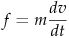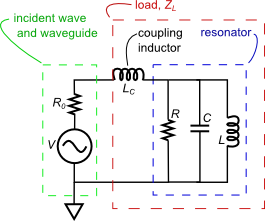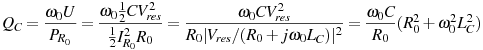There are all sorts of resonances around us, in the world, in our culture, and in our technology. A tidal resonance causes the 55 foot tides in the Bay of Fundy. Mechanical and acoustical resonances and their control are at the center of practically every musical instrument that ever existed. Even our voices and speech are based on controlling the resonances in our throat and mouth. Technology is also a heavy user of resonance. All clocks, radios, televisions, and gps navigating systems use electronic resonators at their very core. Doctors use magnetic resonance imaging or MRI to sense the resonances in atomic nuclei to map the insides of their patients. In spite of the great diversity of resonators, they all share many common properties. In this blog, we will delve into their various aspects. It is hoped that this will serve both the students and professionals who would like to understand more about resonators. I hope all will enjoy the animations.

Origins of Newton's laws of motion

History of mechanical clocks with animations
Understanding a mechanical clock with animations
includes pendulum, balance wheel, and quartz clocks

## Friday, June 7, 2013

### 3.16a Electrical model of the acoustical circuit in Fig. 35 of posting 3.15

 All postings by author previous: 3.16 Introduction and table of contents up: 3.16 Contents of 3.16a - 3.16g next: 3.16b Looking at the resonator part of the circuit

3.16a Electrical model of the acoustical circuit in Fig. 35 of posting 3.15

Keywords: acoustic resonator, coupling iris, modeling, Q0, coupling Q

Topics covered in this posting

• This posting is the first step to mathematically analyze the first of the two resonant scattering animations in an earlier posting.
• In this posting we develop an electrical circuit analog to the acoustical circuit in the original animation.
• We also derive equations for the unloaded Q, i.e. Q0 , and coupling Q, i.e. QC .
1. Modeling the coupling holeFig. 1. Details of the resonator and coupling hole from Fig. 35 of a previous posting. The high velocity region in and near the coupling hole is similar to that of a Helmholtz resonator and has the Helmholtz or breathing mode. On the other hand, because the properties of the Helmholtz mode (resonant frequency, Q, etc.) are so dependent on the details of the hole for the resonance itself (as opposed to just the coupling), we focus on the next mode, the λ/2 mode (which is the first closed pipe mode) that can exist with or without the coupling hole and the hole for this mode mostly affects only the coupling.

At the site of the resonator in Fig. 35 of the previous posting, incident waves travel upwards, hit a wall, and reflect back downwards. A small coupling hole in that wall allows the waves to excite the resonator and after the resonator is excited, for the resonator to radiate waves back out the coupling hole into the waveguide. The incident wave reflection process doubles the acoustic pressure at the wall and thereby doubles the excitation of the resonator.

Waves traveling through the coupling hole see their acoustic velocity concentrated and increased over what they would have had in the waveguide. While the normal parameters to use with a plane wave in an open space are acoustic pressure p and acoustic velocity v, for an acoustic waveguide one uses acoustic pressure and acoustic volumetric velocity u = Av in units of m3/sec and where A is the cross sectional area of the acoustic waveguide. As discussed on Wikipedia the appropriate impedance to use with a waveguide is the characteristic impedance Z = p/Avp/u .

A coupling hole which is short compared with a wavelength acts primarily as a mass element in this acoustical circuit. At first glance, this may seem odd, since there is very little mass of air in the coupling hole compared with that in the wider waveguide. However the coupling hole acts similar to a pinch point (a constriction of lanes) in a traffic jam: it is the sluggishness of the cars in the pinch point, as few as they may be, that impedes the whole traffic flow for miles behind it and determines the throughput of the all these miles of traffic.

We can derive the effective mass (i.e. inductance in the circuit analog) with some simple algebra. Starting with Newton's second law:,
we use the fact that pressure is force divided by area:.    (1)
In the above equation len is the length of the coupling hole and ρ is the mass density (kg/m3) of air in the waveguide. The factor ρ len / A in the above equation plays the same role as an inductance L in circuit analysis (of electrical circuits) in the relation V = L(dI / dt) where the voltage V and current I are the potential and kinetic parameters, respectively.

Thus we model the acoustic circuit in Fig. 35 by the electrical circuit in Fig. 2 below where a coupling inductor LC plays the role that the coupling hole does in Fig. 35. Elmore and Heald (Amazon, Google books) on page 149 explains that the length len of the coupling hole should be extended by a factor 1.64a (where a is the hole's radius) to account for the high velocity region extending slightly past the length of the hole itself. Thus, the inductance to use is:

where K = voltage/(acoustic pressure) is the conversion constant used to convert acoustical pressure into voltage. This conversion constant, although it is arbitrarily chosen, once it is chosen, the one value needs to be consistently used throughout a calculation or modeling.

2. Modeling the acoustic circuit in Fig. 35 and the Q's of this circuitFig. 2. An electrical circuit which is equivalent to the acoustical circuit in Fig. 35.

At the right we see the electrical equivalent of the acoustical circuit of Fig. 35. We see three "legs" protruding out from a circulator in the center. Each leg is a transmission line of characteristic impedance Z0 = R0. The top left leg is connected to the wave source. The waves from this source propagate down the transmission line to port 1 of the circulator which sends them out port 2. At port 2 they travel to the right in the right most transmission line to the resonator. There they are partially absorbed and partially reflected. The reflected waves travel to the left, to the circulator's port 2 and are sent out port 3 to the third leg. In the third leg they travel down and to the left to the terminating absorber having resistance R0, i.e. equal to the characteristic impedance of the transmission lines.

When viewed from the segment of the transmission line between points A and B, Fig. 2  above is similar to Fig. 3  below. The circulator mostly has the function of making sure there is a clean signal from the only source propagating to the right in the section between points A and B. The circuit to the right of point B labelled "load" (consisting of LC and the resonator) has a a certain impedance, which presents a load to the waveguide to the left of point B. For the moment we will label this impedance ZL.

In an upcoming posting (3.21) we will derive an equation for the voltage of a complex load at the end of a transmission line. Using Equation 6 of 3.21, the voltage at point B is given by:

where we have replaced Z0 with R0 because we are assuming the characteristic impedance of the transmission lines is real and equal to R0. We can now draw a simpler equivalent circuit for the waves at point B to replace the more complicated circuit shown in Fig. 2. This simpler circuit is shown in Fig. 3 below. Following (3), we have a voltage source given by V = 2Vsource with a resistor of value R0 in series with V  and in series with the "load" from Fig. 2. We do this because the term in parentheses in (3) is just a voltage divider equation, giving the voltage across ZL when it is in series with a resistor R0.Note that Fig. 3 makes sense in several limits.

• One hypothetical limit is if ZL = R0 so the load ZL perfectly terminates the transmission line and the incident wave is entirely absorbed. Since in this case there is no reflected wave, the total voltage at point B is just the incident signal Vsource, consistent with (3). Note then that in Fig. 3, half the voltage drop from the source will be across R0 and half will be across ZL.
• In the limit that ZL >> R0, then the entire 2Vsource will be across the load, i.e. across ZL. This is consistent with the fact that all the wave will be reflected in this case with zero phase shift and as such the reflected wave will add to the incident wave doubling the amplitude on the load.
• In the limit that ZL → 0, then all the voltage drop in Fig. 3 will be across R0 and none will be across ZL. This is consistent with the fact that all the wave will be reflected but in this case it will have 180 degrees of phase shift and as such the reflected wave will exactly cancel out the incident wave making the total voltage across the load zero.
• These limits are portrayed in the animation in an earlier posting.Fig. 3. The electrical circuit which is equivalent to the circuit of Fig. 2 above, from the point of view of the resonator.

At the left we see the equivalent electrical circuit of the acoustic circuit, from the point of view of the resonator. We model the waveguide and source with an ideal AC voltage source and resistor. The amplitude of the voltage source is twice that of the sound source in Fig. 40 (with whatever conversion factor we wish to use between sound pressure and electronic voltage). That is Vequivalent = 2Vwaveguide. The factor of two allows an open circuit at the end of the waveguide to experience twice the waveguide source voltage (source voltage in an actual waveguide and not this simplified equivalent circuit), while allowing a perfectly matched load resistor at the waveguide end to experience a voltage equal to the actual source voltage.

The waveguide "resistance" R0 is set equal to the equivalent acoustic impedance of the waveguide in Fig. 40.

3. Q's of the above circuitTo calculate Q0 (the intrinsic Q of the resonator alone) and QC (the coupling Q) of our circuit (Fig. 3) we rearrange equation (125) of an earlier posting:

The resonator is modeled as a parallel LRC circuit. The intrinsic Q, i.e. Q0 (the quality factor if the LRC resonator were in a circuit all to itself but magically powered or in free decay) is given by:

where ω0 = 1/√LC   and Vres is the voltage amplitude across the parallel elements of the resonator. The factor of ½ in the numerator is part of the formula for energy in a capacitor, an energy that shuttles between the capacitor and inductor during resonance. The factor of ½ in the denominator is due to time averaging the power loss in the resistor. This power loss oscillates between a minimum value of 0 and maximum value of IRo2R0.

The coupling QC is calculated by assuming that the source voltage is turned off (replaced by a short) and the resonator is magically excited by an ideal voltage source (zero output resistance). The coupling Q equals ω0 times the energy in the resonator divided by the power loss in the coupling resistor:.   (6)Fig. 4. Coupling Q and loaded Q versus the coupling inductor showing the effect the coupling has on these Q's. Both Q's have been divided by Q0 (which equaled 20 in our example) and the coupling inductor was multiplied by the factor ω0/R0 .

As expected, the coupling inductor LC affects the coupling QC. Larger LC means larger QC's and weaker coupling. This corresponds to a smaller coupling hole in the acoustic circuit of Figs. 35 and 1, consistent with (2) remembering that the hole's area A in the denominator of (2) is proportional to the hole's radius squared.

Equation (6) is plotted at the right. On the vertical axis, we plot a normalized coupling QC defined as QC/Q0 , i.e. (6) divided by (5) or QC/Q0 = 1/β = (R0/R)×(1 + x2)  where x = ω0LC/R0 . On the horizontal axis we plot x, the normalized coupling inductance. For the graph we assumed R0/R = 1. To obtain normalized coupling Q's for other R0/R values, multiple the value from the graph by R0/R . We also plotted the normalized loaded Q  i.e. QL/Q0 . Note that a larger coupling inductor mean weaker coupling, a higher coupling Q and higher loaded Q.

In a future posting (3.16c), we calculate the steady state equations for this equivalent circuit above and graph the results. There, we shall see that the resonant frequency is given by ω0 = 1/√LC   for high loaded Q's but at lower Q's the resonant frequency is affected by the coupling inductor LC .

Before we get to the entire circuit, we first examine just the resonator part in the next posting.

 All postings by author previous: 3.16 Introduction and table of contents up: 3.16 Contents of 3.16a - 3.16g next: 3.16b Looking at the resonator part of the circuit